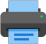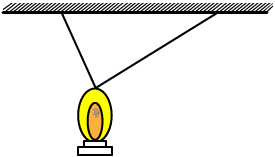Lista de exercícios do ensino médio para impressãoSelect all.
print selected exercises
Knowing that in the right triangle ABC shown in the picture b = 1 and c = 2, find x.
a)
$\,\sqrt{2}\,$
b)
$\,\dfrac{3}{2}\,$
c)
$\,\dfrac{3\sqrt{2}}{2}\,$
d)
$\,\dfrac{2}{3}\,$
e)
$\,\dfrac{2\sqrt{2}}{3}\,$×
The measure of the two legs of the right triangle T are, respectively, 12 cm and 5 cm each, so the length of the altitude to the hypotenuse of T is:
a)
$\,\dfrac{12}{5}\,$ cm
b)
$\,\dfrac{5}{13}\,$ cm
c)
$\,\dfrac{12}{13}\,$ cm
d)
$\,\dfrac{25}{13}\,$ cm
e)
$\,\dfrac{60}{13}\,$ cm

×
In the picture below, ABFG and BCDE are squares and their each one sides measures, respectively, a and b. Knowing that $\;\overline{AG}\,=\,\overline{CD}\,+\,2\;\,$ and the perimeter of the triangle ACG is 12, we can assure that a e b are both inside the interval:
a)
]1; 5[
b)
]0; 4[
c)
]2; 6[
d)
]3; 7[
e)
]4; 8[×
If the length of the legs of a right triangle are $\,\sqrt{3}\;$ and $\;\sqrt{4}\,$, the length of the hypotenuse is:
a)
$\,\sqrt{5}\,$
b)
$\,\sqrt{7}\,$
c)
$\,\sqrt{8}\,$
d)
$\,\sqrt{9}\,$
e)
$\,\sqrt{12}\,$

×
The lantern shown in the picture is suspended by two strings perpendicular to each other. Knowing the length of each string is 1/2 and 6/5 , respectively, what is the distance between the lantern and the ceiling?
a)
1,69
b)
1,3
c)
0,6
d)
1/2
e)
6/13